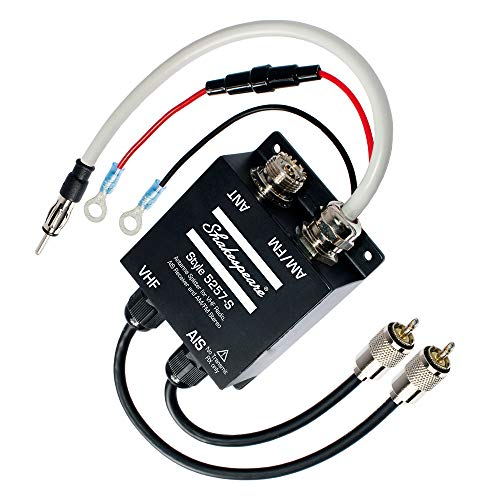# RF Splitter Calculator

An RF Splitter (also known as a power divider) is used to split the input signal into 2 or more equally powered signals. This tool calculates the total loss in dB of the signal on any of the output ports. It also gives the output signal level in dBm.

Input the following:

• Number of ports
• Input Signal (dBm)

🔁 RF Power Combiner

## Background

RF splitters are used to split an antenna output for instance into two receivers. They can also be used to split the output of a signal generator into two.

## Formula

Loss (dB) = 10*Log10(N) + IL

where

• N is the number of paths
• IL is the insertion loss

## Example Calculation

A four way splitter with an insertion loss of 0.5 dB has a total path loss of 6.52 dB on any of the outputs. This means that a -20 dBm input signal will be -26.52 dBm at all of the output ports.

## Number of Splitter Outputs

As the number of output ports increases, so also does the total path loss. A signal output from a two-way splitter is stronger than that from a three-way splitter.

💡 This is something to consider when purchasing a device for splitting the signal from your antenna – don’t buy a three port device if you only have two TVs. The output power will be weaker as a result of the extra port.

## Can a Power Splitter be used as a Combiner?

Splitters are generally passive devices as in they don’t require DC power for operation. A passive splitter can be used as a combiner when reversed. However, some splitters (such as the three-way device below) contain amplifiers and therefore require DC power in order to operate. These splitters cannot be used as combiners.